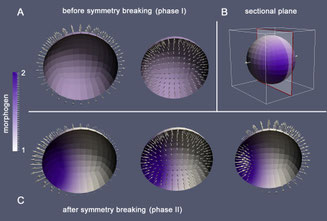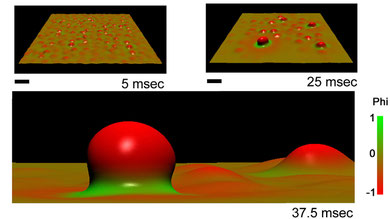## Mathematical techniques

Applied analysis: Continuous models, such as Partial differential equations (PDEs), especially nonlinear coupled PDE systems of higher order, calculus of variations, Helfrich- and Cahn-Hilliard theory, geometric PDEs

Mathematical modeling: Biomechanical and biochemical modeling, energy based models, Gradient flow, multiscale models (combining continuous models with dissipative particle dynamics (DPD)), elasticity theory, continuum mechanics: Viscoelastic and hyperelastic models, fluid-structure interactions

Numerics/Simulation tools: Parametric Finite element methods (FEM) using Gascoigne 3D

Biostatistics: statistical ecology, non-parametric methodsFinite Element Simulation of pattern formation in biological tissue

## Biological model systems:

Biomembranes: Chemical and mechanical pattern formation in lipid bilayers with associated membrane proteins

Biotissues: Pattern formation and shape generation in biological tissues with morphogensFinite Element Simulation of pattern formation in biological membranes

## Biological applications:

ESCRT: Bud formation and cargo-sorting induced by molecules belongin to the "endosomal sorting complex required for transport" (ESCRT) family.

Hydra: Early pattern formation and axis defining processes in the freshwater polyp Hydra

Hydroids: Mecanical aspects of growth-pulsations in different hydroids

Ecology: population development and interaction fusing biostatistics with mathematival modeling